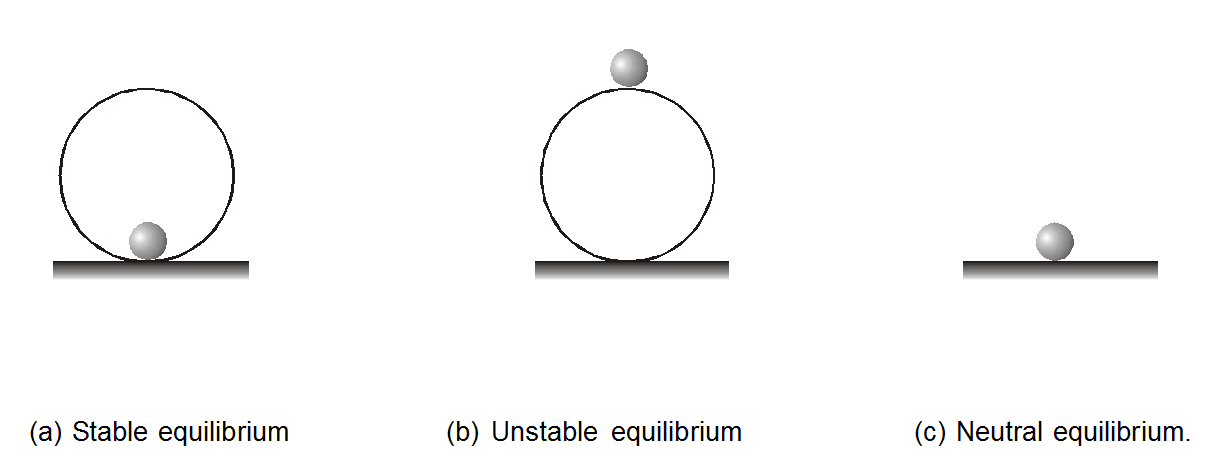Most Affordable JEE | NEET | 8,9,10 Preparation by Kota's Top IITian Doctor Faculties

# Types of Equilibrium - What is Equilibrium? - eSaral`

Hey, Do you want to learn about the Types of Equilibrium? If so. Then you are at the right place.

## What is Equilibrium?

A body is said to be in translatory equilibrium if the net force acting on the body is zero, $\overrightarrow{\mathrm{F}}_{\text {net }}=0$

If the force are conservative then $\mathrm{F}=-\frac{\mathrm{dU}}{\mathrm{dr}}$

For equilibrium F = 0.

So, $-\frac{d U}{d r}=0$

Or

$\frac{\mathrm{dU}}{\mathrm{dr}}=0$

At equilibrium position slope of U-r graph is zero or the potential energy is optimum (maximum or minimum or constant).

## Types of Equilibrium.

1. Stable Equilibrium
2. Unstable Equilibrium
3. Neutral Equilibrium

### Stable Equilibrium:

When a particle is slightly displaced from equilibrium and it tends to come back towards equilibrium then it is said to be in stable equilibrium

### Unstable Equilibrium:

When a particle is displaced from equilibrium and it tends to move away from equilibrium position then it is said to be in unstable equilibrium

### Neutral Equilibrium:

When a particle is displaced from equilibrium and no force acts on it then the equilibrium is said to be neutral equilibrium

The situation where F = 0 and $\frac{\mathrm{d} \mathrm{U}}{\mathrm{dr}}=0$ can be obtained under three conditions.Three identical balls are placed in equilibrium in positions as shown in fig. (a), (b), and (c) respectively.

1. The ball is placed inside a smooth spherical shell. This ball is in a stable equilibrium position.
2. The ball is placed over a smooth sphere. This is in the Unstable equilibrium position.
3. The ball is placed on smooth horizontal ground. This ball is in the Neutral equilibrium position.

## Difference Between Stable, Unstable, and Neutral Equilibrium

For a better understanding of this chapter, please check the detailed notes of the Work Energy and Power. To watch Free Learning Videos on physics by Saransh Gupta sir Install the eSaral App.

Op.innocent sane
March 23, 2023, 6:35 a.m.
Lets like,share and subscribe our knowledge from one person to another for a better future
Suzan
Dec. 6, 2022, 7:10 p.m.
this info has helped me to increase on my on my vision on types of equilibrium
saviour
July 17, 2022, 9:17 p.m.